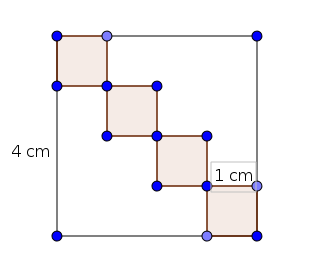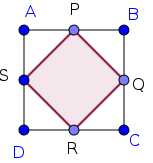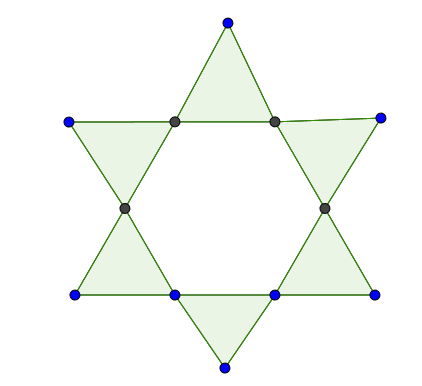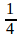Question Pool Mathematics of Chance

# Mathematics of Chance - SAMAGRA Question Pool & Answers | Class 10 English Medium

Kerala Syllabus SAMAGRA SCERT SAMAGRA Question Pool for Class 10 English Medium Maths Mathematics of ChanceQn 1.

In class 10A there are 20 boys and 15 girls .In 10B there are 15 boys and 25 girls. One student from each class is to be selected for a competition. What is the probability of

a) both are boys?

b) at least one girl?

Get Free Study Materials + 1 Week Free Trial of BrainsPrep Class 10 English Medium Tuition

Qn 2.

Without looking if we put a dot in the figure,  what is the probability that the dot being in the shaded portion ?Get Free Study Materials + 1 Week Free Trial of BrainsPrep Class 10 English Medium Tuition

Qn 3.

Ask somebody to say a two digit number.

a) What is the probability of being the number 10?

b) What is the probability that the number being a perfect square?

Get Free Study Materials + 1 Week Free Trial of BrainsPrep Class 10 English Medium Tuition

Qn 4.

In a box there are 12 black beads and some white beads. One bead is selecting randomly. The probability of getting a white bead is 1/3 then

what is the probability of getting a black bead?

How many beads are there in the box?

Get Free Study Materials + 1 Week Free Trial of BrainsPrep Class 10 English Medium Tuition

Qn 5.

In a bag there are 6 red balls and 4 white balls and in another box there are 5 red balls and 6 white balls.

a) What is the probability of taking a white ball from the first bag?

b) Which bag has more probability of getting a red ball?

Get Free Study Materials + 1 Week Free Trial of BrainsPrep Class 10 English Medium Tuition

Qn 6.

In the figure , ABCD is a square and P,Q,R,and S are the midpoints of its sides.Without looking into it, if we put a dot ,what is the chance that the dot is in the shaded portion?

Get Free Study Materials + 1 Week Free Trial of BrainsPrep Class 10 English Medium Tuition

Qn 7.

A box contains 12 white beads,10 red beads,and 8 blue beads. Without looking ,if one bead is taken , what is the chance of it

b) being a blue or white bead?

Get Free Study Materials + 1 Week Free Trial of BrainsPrep Class 10 English Medium Tuition

Qn 8.

In a box there are 20 red ink pens 50 blue ink pens and 30 black ink pens. One pen is taking at random from the box.

a) What is the probability of getting a black ink pen?

b) What is the probability of getting a black ink or blue ink pen?

c) What is the probability of not getting a red ink pen?

Get Free Study Materials + 1 Week Free Trial of BrainsPrep Class 10 English Medium Tuition

Qn 9.Equilateral triangles are drawn on each side of a regular hexagon. if we put a dot in the figure what is the probability that the dot being in the shaded portion

Get Free Study Materials + 1 Week Free Trial of BrainsPrep Class 10 English Medium Tuition

Qn 10.

Numbers from 1 to 10 are written in paper slips and put in to a bag and numbers 5,10,15,in another bag. If one number is taken from each bag without looking into it

a) write the pairs in which both are even numbers?

b)write the probability of getting at least one odd number?

Get Free Study Materials + 1 Week Free Trial of BrainsPrep Class 10 English Medium Tuition

Qn 11.

A box contains slips numbered 1,2,3,4. Another box contains slips numbered 1,2,3. If one slip is taken from each,

a) Which will be the smallest sum of the numbers ?

b) What is the probability of getting the sum 6 ?

Get Free Study Materials + 1 Week Free Trial of BrainsPrep Class 10 English Medium Tuition

Qn 12.

There are 25 ripe and 15 raw mangoes in a box. Raju  randomly selected a mango. After that Fazil is selecting a mango from the box.

a) What is the probability to select a ripe mango by Raju ?

b) What is the probability to select a ripe mango by Fazil ?

Get Free Study Materials + 1 Week Free Trial of BrainsPrep Class 10 English Medium Tuition

Qn 13.

There are 36 beads in a box, some are white and some are black. The probability of drawing a white bead isa) What is the probability of drawing a black bead?

b) How many black beads are there in the box?

Get Free Study Materials + 1 Week Free Trial of BrainsPrep Class 10 English Medium Tuition

Qn 14.

Each of  the 11 letters of the word MATHEMATICS  is written on separate cards and put into a box. If we take one card randomly from it,

a) What is the probability of getting the letter 'M'?

b) What is the probability of getting a vowel?

Get Free Study Materials + 1 Week Free Trial of BrainsPrep Class 10 English Medium Tuition

Qn 15.

In a box there are 20 ripen mangoes and some raw mangoes ,without looking into it if we take a mango from the box the probability of getting a raw mango is twice the probability of getting a ripen mango. How many raw mangoes are there in the box?

Probability of getting raw mango is twice the probability of getting ripen mango

So no. of raw mango = 2 X no. of ripen mangoes

Get Free Study Materials + 1 Week Free Trial of BrainsPrep Class 10 English Medium Tuition

Qn 16.

There are 21 blue buttons and 29 white buttons in a bag. Without looking into the bag, a button is taking randomly

a) write the probability for getting a blue button.

b)write the probability for getting a white button.

c) which button has more chance?

Get Free Study Materials + 1 Week Free Trial of BrainsPrep Class 10 English Medium Tuition

Qn 17.

What is the probability that 5 Saturdays in the month of  February may occur in a leap year ?

Get Free Study Materials + 1 Week Free Trial of BrainsPrep Class 10 English Medium Tuition